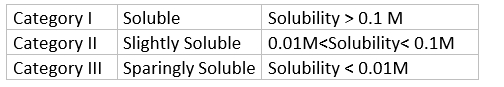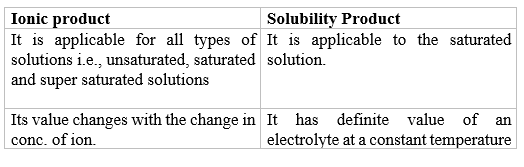# Solubility Equlibrium And Solubility Product

## Solubility Equlibrium

• The solubility of salts depends on many factors . The important one among them is lattice enthalpy of the salt and the solvation enthalpy of the ions in a solution
• The solvation enthalpy of the salt must be greater than its lattice enthalpy to disolve in the solvent
• The amount of solvation enthalpy depends on the nature of the solvent.
• non-polar (covalent) solvent, solvation enthalpy is small,So salt does not dissolve in it
• Each salt has its characteristic solubility which depends on temperature
• we can classify salats based on Solubility## Solubility Products

It is applicable to sparingly soluble salts. There is equilibrium betwenb ions and unionsed solid substance. It is defined as the product of solubility of ions raised to the power equal to the no of times each ion occur in the equation for solubility's.
Lets take an example of below reaction
$BaSO_4(s) \rightleftharpoons Ba^{2+} (aq) + SO_4^{2–}(aq)$
The equilibrium constant is given by the equation:
$K = \frac {[Ba^{2+}][SO_4^{2–}]} { [BaSO_4]}$
For a pure solid substance the concentration remains constant and we can write
$K_{sp} = K[BaSO4] = [Ba^{2+}][SO4^{2–}]$
Ksp the solubility product constant or simply solubility product. The concentrations of the two ions will be equal to
the molar solubility of the barium sulphate. If molar solubility is S
$K_{sp} =S^2$
Examples
(a)$AgCl \rightleftharpoons Ag+ + Cl-$
$K_{sp} = [S]^1 \times [S]^1$
$= S^2$
(b) $(CH_3COO))2 Mg \rightleftharpoons Mg^{+2} + 2CH3COO^-$
$K_{sp} = 4S^3$
(c) $Pb Cl2 \rightleftharpoons Pb^{+2} + Cl^-$
$K_{sp} =[S] \times [2S]^2$
$K_{sp} = 4S^3$
(d) $Ca_3 (PO_4)_2 \rightleftharpoons 3Ca^{+2} + 2PO_4^{-3}$
$K_{sp} = (3S)^3 (2S)^2=108S^5$

In General
$M_xX_y(s) \rightleftharpoons xM^{p+}(aq) + yX^{q–} (aq)$
(where $x \times p+ = y \times q–$)
If S is the Molar solubilty of the substance then
$Ksp = [Mp+]^x[Xq– ]^y$
$= (xS)^x (yS)^y =x^x y^y S^{x+y}$

## Difference between Ionic Product and Solubility Product## Common Ion Effect on Solubility of Ionic Salts

(i) When Ksp = ionic product ,solution is saturated in which no more solute can be dissolved.
(ii) Ksp > ionic product,Solution is unsaturated which more solute can be dissolved.
(iii) Ksp < ionic product , Solution is super saturated and precipitation takes place.
if we take a saturated solution of sodium chloride and pass HCl gas through it, then sodium chloride is precipitated due to increased concentration
of chloride ion available from the dissociation of HCl

## Example

Question 1
Give the solubility of $PbCl_2$ if $K_{sp}$ for PbCl2 is $4 \times\ {10}^{-12}$.
Solution
$PbCl2 \rightleftharpoons Pb^{+2} + 2Cl^{-}$
$K_{sp}=[S] \times [2S]^2=4S^3$
$4 \times\ {10}^{-12} = 4S^3$
${10}^{-12} = S^3$
$S=10^{-4}$

Question 2
0.03 mole of $Ca^{+2}$ ions is added to a litre of 0.01 M $SO_4^{-2}$ Solution will it cause precipitation of CaSO4 (Ksp of CaSO4 = $2.4 \times\ {10}^{-5}$)
Solution
$CaSO_4 \rightleftharpoons Ca^{+2} + SO_4^{-2}$
Ionic product of the two ions:-
$= [Ca+2] \times [SO4-2]= \left[\frac{0.03}{1}\right]\ \times\left[\frac{0.01}{1}\right]= 3 \times\ {10}^{-4}$
Since IP > Ksp
Therefore Precipitate will form